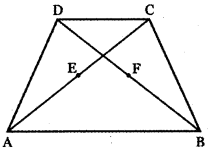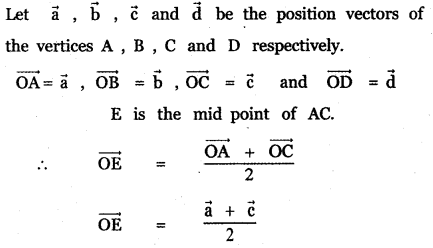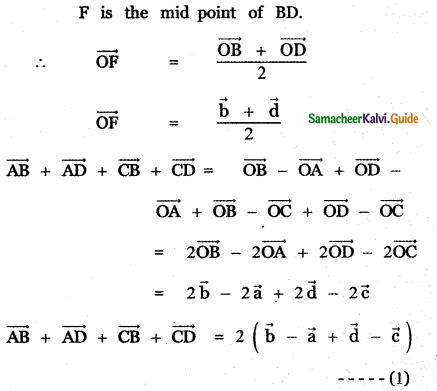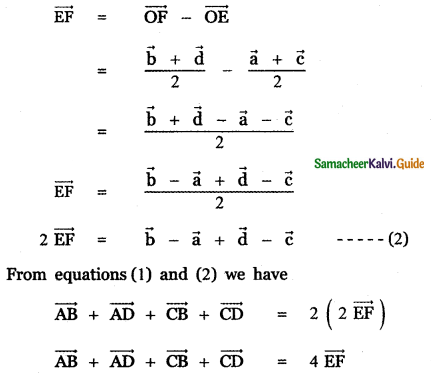Tamilnadu State Board New Syllabus Samacheer Kalvi 11th Maths Guide Pdf Chapter 8 Vector Algebra – I Ex 8.1 Text Book Back Questions and Answers, Notes.

## Tamilnadu Samacheer Kalvi 11th Maths Solutions Chapter 8 Vector Algebra – I Ex 8.1

Question 1.
Represent graphically the displacement of
(i) 45cm, 30° north of east
(ii) 80 km, 60° south of west
(i) 45cm, 30° north of east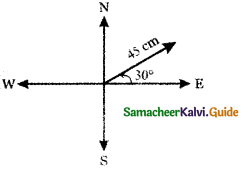(ii) 80 km, 60° south of west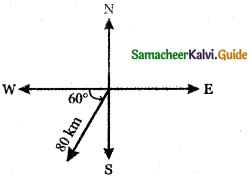Question 2.
Prove that the relation R defined on the set V of all vectors by ‘$$\vec{a}$$ R $$\vec{b}$$ if $$\vec{a}$$ = $$\vec{b}$$’ is an equivalence relation on V.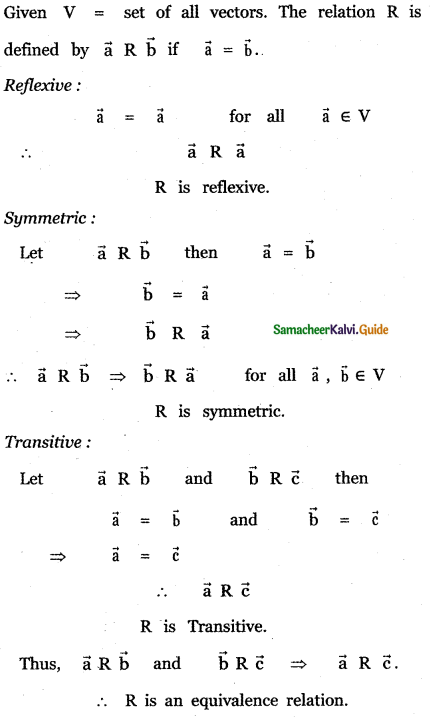Question 3.
Let $$\vec{a}$$ and $$\vec{b}$$ be the position vectors of the points A and B. Prove that the position vectors of the points which trisects the line segment AB are $$\frac{\overrightarrow{\mathbf{a}}+2 \overrightarrow{\mathbf{b}}}{3}$$ and $$\frac{\overrightarrow{\mathbf{b}}+2 \overrightarrow{\mathbf{a}}}{3}$$.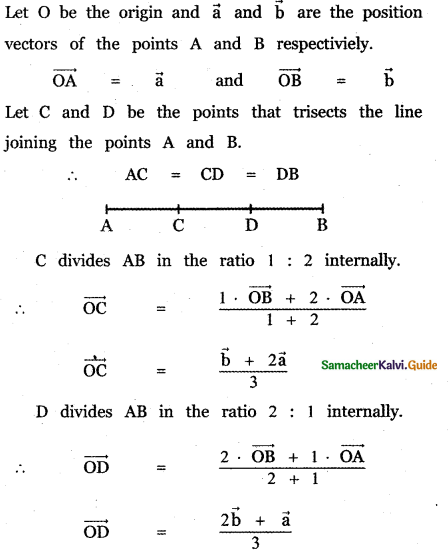Question 4.
If D and E are the midpoints of the sides AB and AC of a triangle ABC, prove that $$\overrightarrow{\mathbf{B E}}$$ and $$\overrightarrow{\mathbf{D C}}$$ = $$\frac{3}{2} \overrightarrow{\mathrm{BC}}$$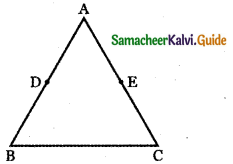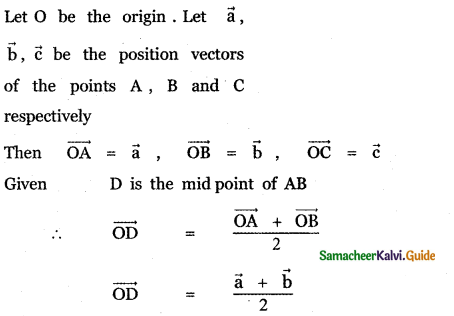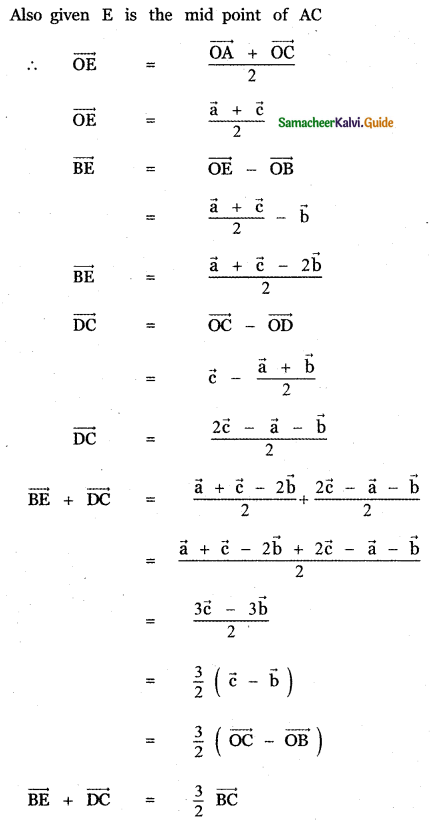Question 5.
Prove that the line segment joining the midpoints of two sides of a triangle is parallel to the third side whose length is half of the length of the third side.
ABC be the triangle and D, E, F is the midpoints of the sides AB, BC, and AC respectively.
Let O be the origin and let $$\vec{a}$$, $$\vec{b}$$ and $$\vec{c}$$ are the position vectors of the points A, B and C respectively.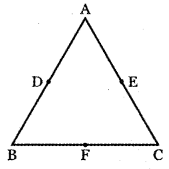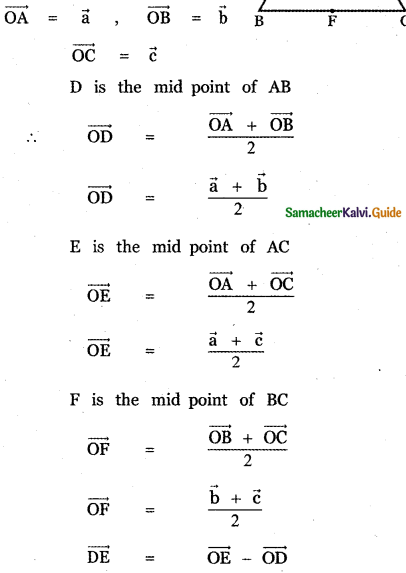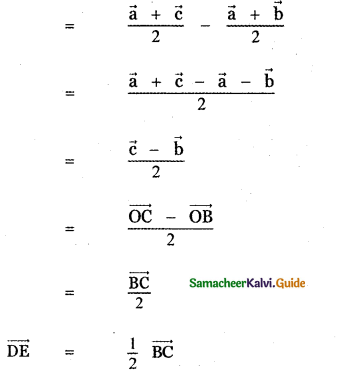∴ $$\overrightarrow{\mathbf{D E}}$$ is parallel to $$\overrightarrow{\mathbf{B C}}$$ and length of DE is half the length of BC. Similarly we can prove $$\overrightarrow{\mathbf{E F}}$$ = $$\frac{1}{2} \overrightarrow{\mathrm{AB}} \cdot \overrightarrow{\mathrm{EF}}$$
parallel to $$\overrightarrow{\mathbf{A B}}$$ and length of E is half the length of AB.
Also $$\overrightarrow{\mathbf{D F}}$$ = $$\frac{1}{2} \overrightarrow{\mathrm{AC}}$$

$$\overrightarrow{\mathbf{D F}}$$ is parallel to $$\overrightarrow{\mathbf{A C}}$$ and length of DF is half the length of AC . Thus, the line segment joining the mid points of two sides of a triangle is parallel to the third side whose length is half of the length of the third side.Question 6.
Prove that the line segments joining the midpoints of the adjacent sides of a quadrilateral form a parallelogram.
Let ABCD be any quadrilateral D, E, F, G are the midpoints of the sides AB, BC, CD, AD respectively.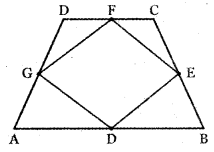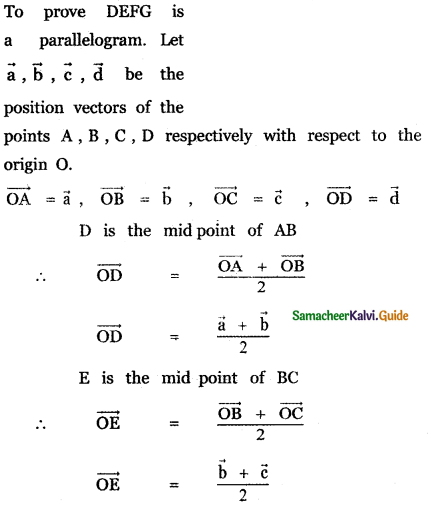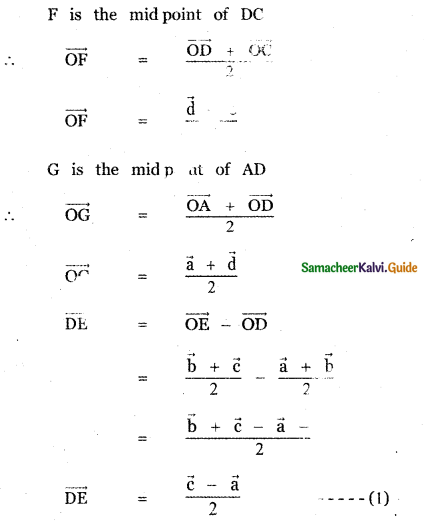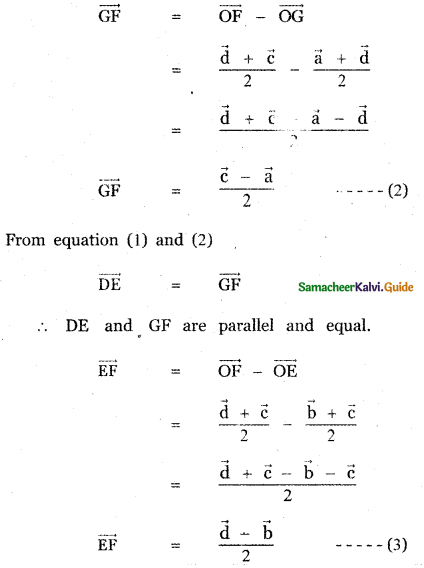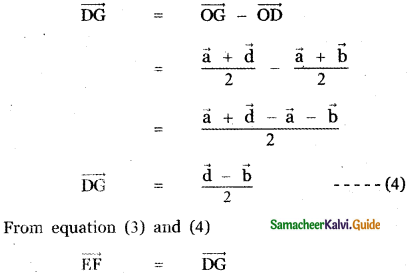∴ EF and DG are parallel and equal.
∴ In the quadrilateral, DEFG’s opposite sides are parallel and equal. Therefore, DEFG is a parallelogram.Question 7.
If $$\vec{a}$$ and $$\vec{b}$$ represent a side and a diagonal of a parallelogram , find the other sides and the other diagonal.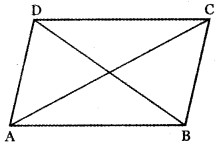Let ABCD be a parallelogram
Let $$\overrightarrow{\mathbf{A B}}$$ = $$\vec{a}$$
$$\overrightarrow{\mathbf{A C}}$$ = $$\vec{b}$$
Since ABCD is a parallelogram , we have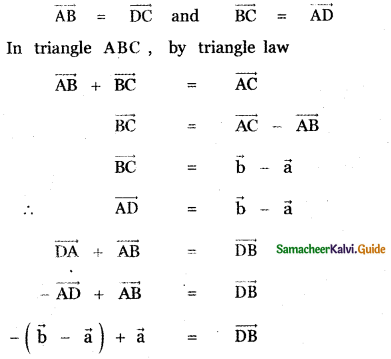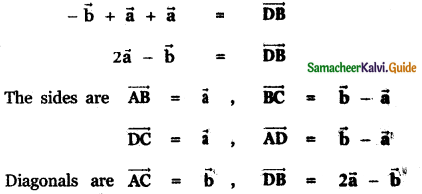Question 8.
If $$\overline{\mathbf{P O}}+\overline{\mathbf{O Q}}$$ = $$\overline{\mathbf{Q O}}+\overline{\mathbf{O R}}$$, prove that the points P , Q , R are collinear.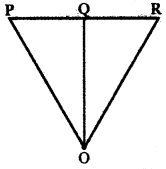Let P, Q , R be the given points . Let O be the origin. Then the position vectors of
P, Q, R are $$\overrightarrow{\mathrm{OP}}$$, $$\overrightarrow{\mathrm{OQ}}$$ and $$\overrightarrow{\mathrm{OR}}$$.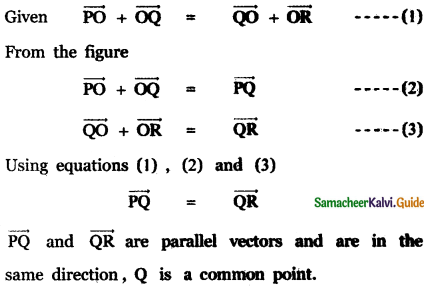∴ P, Q, R lie on a straight line.
Hence, P, Q, R are collinear.Question 9.
If D is the midpoint of the aide BC of i triangle ABC , prove that $$\overrightarrow{\mathbf{A B}}$$ + $$\overrightarrow{\mathbf{A C}}$$ = 2 $$\overrightarrow{\mathbf{A D}}$$
Given D is the mid point of the side BC of a triangle ABC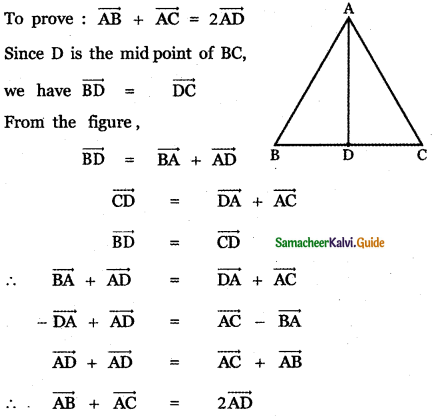Question 10.
If G is the centroid of a triangle ABC, prove that $$\overrightarrow{\mathbf{G A}}+\overrightarrow{\mathbf{G B}}+\overrightarrow{\mathbf{G C}}=\overrightarrow{\mathbf{0}}$$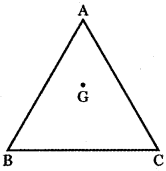Let ABC be the triangle with centroid G. Let $$\vec{a}$$, $$\vec{b}$$ and $$\vec{c}$$ be the position vectors of the vertices A, B and C respectively with respect to the origin O. Then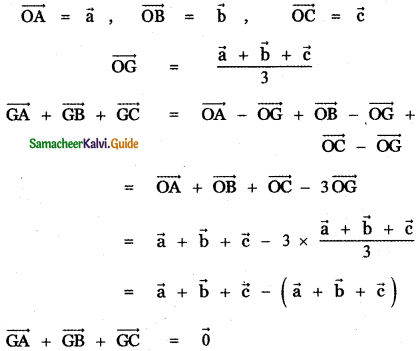Question 11.
Let A, B, and C be the vertices of a triangle. Let D, E, and F be the midpoints of the sides BC, CA, and AB respectively. Show that $$\overrightarrow{\mathbf{A D}}+\overrightarrow{\mathbf{B E}}+\overrightarrow{\mathbf{C F}}=\overrightarrow{\mathbf{0}}$$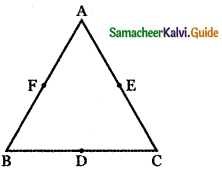Let ABC be the triangle. D, E, F are the mid points of the sides BC , CA , AB respectively . Let $$\vec{a}$$, $$\vec{b}$$ and $$\vec{c}$$ be the position vectors of the vertices A, B, C respectively. Then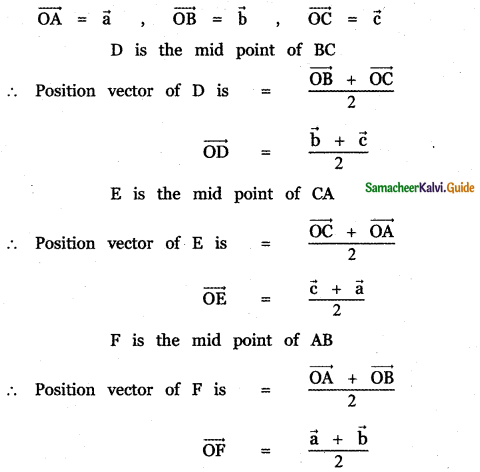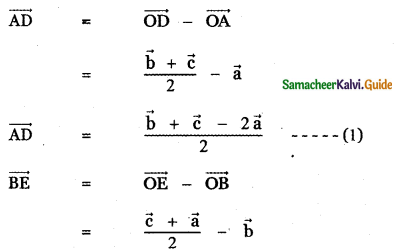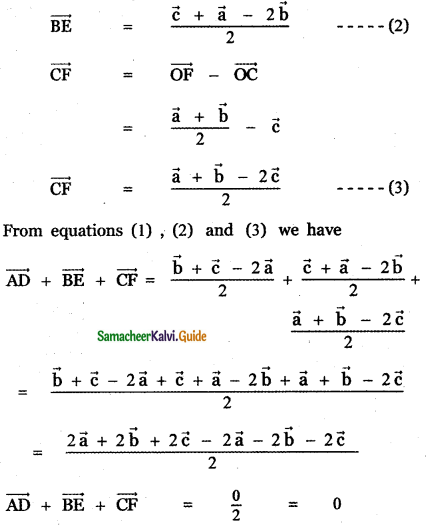Question 12.
If ABCD is a quadrilateral and E and F are the midpoints of AC and BD respectively, then Prove that $$\overrightarrow{\mathbf{A B}}+\overrightarrow{\mathbf{A D}}+\overrightarrow{\mathbf{C B}}+\overrightarrow{\mathbf{C D}}=4 \overrightarrow{\mathbf{E F}}$$